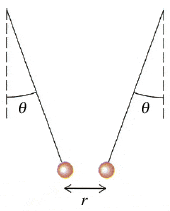# Electric Forces between charges

• thst1003

#### thst10031.

Hanging from threads are two charged balls made of pith (a very light, spongy material that comes from inside the stems of certain plants). The balls each have a mass of 0.100 g and a charge of the same magnitude q and are attracted toward each other, as shown in the figure below. (Take θ = 18.9° and r = 1.82 cm.)

Question: What is the magnitude of the electric force?

F = k (q x q/r^2)